Processing ......FreeComputerBooks.com Links to Free Computer, Mathematics, Technical Books all over the World

P, NP, and NP-Completeness: The Basics of Computational Complexity
🌠 Top Free JavaScript Books - 100% Free or Open Source!
• Title: P, NP, and NP-Completeness: The Basics of Computational Complexity
• Author(s) Oded Goldreich
• Publisher: Cambridge University Press; 1 edition (August 16, 2010)
• Paperback: 214 pages
• eBook: HTML and PDF (375 pages)
• Language: English
• ISBN-10: 0521122546
• ISBN-13: 978-0521122542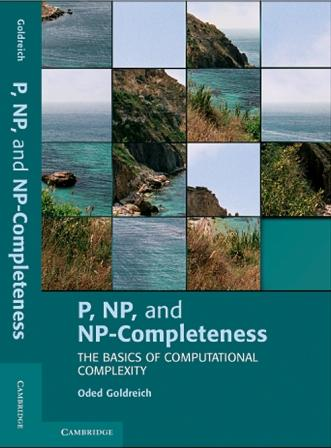Book Description

This undergraduate introduction to computational complexity offers a wide perspective on two central issues in theoretical computer science. The book starts with the relevant background in computability, including Turing machines, search and decision problems, algorithms, circuits, and complexity classes, and then focuses on the P-versus-NP Question and the theory of NP-completeness.

• Oded Goldreich is a professor of Computer Science at the Faculty of Mathematics and Computer Science of Weizmann Institute of Science, Israel. His research interests lie within the theory of computation and are, specifically, the interplay of randomness and computation, the foundations of cryptography, and computational complexity theory. He won the Knuth Prize in 2017.
Reviews, Ratings, and Recommendations: Related Book Categories: Read and Download Links:Similar Books:
•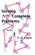Solving NP-Complete Problems (Forbes D. Lewis)

This is a textbook on heuristic algorithms. From the table of contents: Classes of Problems; Integer Programming; Enumeration Techniques; Dynamic Programming; Approximate Solutions; Local Optimization; Natural Models.

•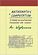Mathematics and Computation (Avi Wigderson)

This book provides a broad, conceptual overview of computational complexity theory - the mathematical study of efficient computation. With important practical applications to computer science and industry, computational complexity theory, etc.

•Think Complexity: Science and Modeling, 2nd Ed. (Allen Downey)

This book is about complexity science, data structures and algorithms, intermediate programming in Python, and the philosophy of science. It presents features that make Python such a simple and powerful language. Code with a solution for each exercise.

•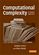Complexity Theory: A Modern Approach (Sanjeev Arora, et al)

This beginning graduate textbook describes both recent achievements and classical results of computational complexity theory, including interactive proofs, PCP, derandomization, and quantum computation.

•Algorithms and Complexity (Herbert S. Wilf)

This is an introductory book on the design and analysis of algorithms. The author uses a careful selection of a few topics to illustrate the tools for algorithm analysis.

•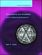Computability and Complexity: From a Programming Perspective

This book is an introduction to the basic concepts of computability, complex, and the theory of programming languages. Its goal is to build a bridge between computability and complexity theory and other areas of computer science, especially programming.

•Quantum Computing Since Democritus (Scott Aaronson)

This book takes readers on a tour through some of the deepest ideas of maths, computer science and physics. Full of insights, arguments and philosophical perspectives, the book covers an amazing array of topics.

•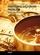Traveling Salesman Problem, Theory and Applications

This book is a collection of current research in the application of evolutionary algorithms and other optimal algorithms to solving the TSP problem, including Artificial Immune Systems, Genetic Algorithms, Neural Networks and Differential Evolution Algorithm.

•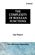The Complexity of Boolean Functions (Ingo Wegener)

Initially deals with the wee-known computation models, and goes on to special types of circuits, parallel computers, and branching programs. It presents a large number of recent research results f Boolean functions previously unavailable in book form.

•Computational Complexity (Wikibooks)

This book contains material that should be core knowledge in the theory of computation for all graduates in computer science. It starts with classical computability theory which forms the basis for complexity theory.

•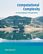Computational Complexity: A Conceptual Perspective (Goldreich)

This book offers a conceptual introduction to the study of the intrinsic complexity of computational tasks. It is intended to serve advanced undergraduate and graduate students, either as a textbook or for self-study.

Book Categories
 :All CategoriesTop Free BooksRecent BooksMiscellaneous BooksComputer EngineeringComputer LanguagesComputer ScienceData Science/DatabasesJava and Java EE (J2EE)Linux and UnixMathematicsMicrosoft and .NETMobile ComputingNetworking and CommunicationsSoftware EngineeringSpecial TopicsWeb Programming
Other Categories